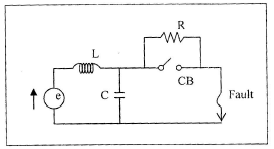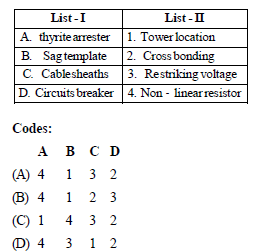# Test: Power Systems - 8

## 25 Questions MCQ Test Electrical Engineering SSC JE (Technical) | Test: Power Systems - 8

Description
Attempt Test: Power Systems - 8 | 25 questions in 50 minutes | Mock test for Electrical Engineering (EE) preparation | Free important questions MCQ to study Electrical Engineering SSC JE (Technical) for Electrical Engineering (EE) Exam | Download free PDF with solutions
QUESTION: 1

Solution:
QUESTION: 2

Solution:
QUESTION: 3

### In connection with arc the extinction in circuit breaker, resistance switching is employed where in a resistance is placed in parallel with the poles of the circuit breaker as shown in the figure. This process introduces damping in the LC circuit. For critical damping, the value of 'R' should be equal toSolution:
QUESTION: 4

Consider the following moderators used in nuclear reactors:
1. Graphite 2. Beryllium
3. Heavy water
Their correct sequence in increasing order of their neutron absorption cross sections is

Solution:
QUESTION: 5

Match List-I with List-II and select the correct answer using the given below the lists:Solution:
QUESTION: 6

lighting arrestor connected between the line and earth in a power system

Solution:

Lightning arresters or surge diverters:

• A lightning arrester or a surge diverter is a protective device which conducts the high voltage surges on the power system to the ground
• It consists of a spark gap in series with a non-linear resistor
• The length of the gap is so set that normal line voltage is not enough to cause an arc across the gap, but a dangerously high voltage will break down the air insulation and form an arc
• The property of the non-linear resistance is that its resistance decreases as the voltage (or current) increases and vice-versa
• One end of the diverter is connected to the terminal of the equipment to be protected and the other end is effectively grounded
QUESTION: 7

Corona loss can be reduced by the use of follow conductors, because

Solution:
QUESTION: 8

The insulation of modern EHV lines is designed based on

Solution:
QUESTION: 9

While using air-blast circuit beaker, current chopping is a phenomenon often observed when

Solution:
QUESTION: 10

In a power station the cost of generation of power reduces most effectively when

Solution:
QUESTION: 11

Shunt compensation in an EHV line is used to

Solution:
QUESTION: 12

In pumped storage scheme, the generator is also used us

Solution:
QUESTION: 13

Consider the following statement: Distance relays used for protection of transmission line might operate undesirable due to
1. magnetizing-current in rush
2. Power swings
3. Series compensating capacitors
4. Switching over voltage
Of these statements

Solution:
QUESTION: 14

The number of discs in a string of insulators for 400 kV ac overhead transmission line lies in the range of

Solution:
QUESTION: 15

The insulation resistance of a single -core cable is 200 MW/ km.The insulation resistance for 5 km length is

Solution:
QUESTION: 16

Consider the following statements:
1. By using bundle conductors is an overhead line, the corona loss is reduced
2. By using bundle conductors, the inductance of transmission line increase and capacitance reduces.
3. Corona loss causes interference in adjoining communication lines.
What of these statement are correct ?

Solution:
QUESTION: 17

Which one of the following sequences of operation represents the rated operating duty cycle of a circuit breaker? (O-open; C-close; t= 3 sec; T=3min)

Solution:
QUESTION: 18

A2-phase circuit breaker is rated at 2000 MVA. 33kV; its making current will be

Solution:
QUESTION: 19

The most accurate and versatile method of achieving reactive power compensation is by using

Solution:
QUESTION: 20

If the positive, negative and zero-sequence reactance's of an element of a power system are 0.3, 0.3 and 0.8 respectively, then the element would be a

Solution:
QUESTION: 21

Disruptive corona begins in smooth cylindrical conductors in air at NTP if the electric field intensity at the conductor surface goes up to

Solution:
QUESTION: 22

Load flow studies involve solving simultaneous

Solution:
QUESTION: 23

If the inductance and capacitance of power system are respectively 1 H and 0.01mF and the instantaneous value of interrupted current is 10 A, then the voltage across the breaker contact will be

Solution:
QUESTION: 24

For successful parallel operation of two singlephase transformers, the most essential condition is that their

Solution:
QUESTION: 25

Arrange the following in the correct sequence in which the flue gas passes through them after coming out of the boiler in thermal power station:

1. I.D. Fan.

2. Air Preheater

3. Economizer

4. Electrostatic precipitator

Select the correct answer using the codes given below:Codes:

Solution:Use Code STAYHOME200 and get INR 200 additional OFF Use Coupon Code